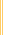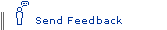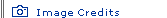# The Power of Tiny ThingsEinstein's Big Idea homepage

 This feature requires Flash, a free software plug-in, and JavaScript. Install Flash, or go to the printable version.Have you ever thought about the power of a paper clip? If you could convert the mass of a paper clip entirely to energy, how big a punch would it pack? Einstein's most famous equation, E = mc2, indicates the calculation is straightforward: the energy released would be equivalent to the mass times the speed of light squared. Yet the answer is far from intuitive because, for one thing, the speed of light is immense—670 million mph—making the speed of light squared almost inconceivable. In this quiz, discover the answer and explore other examples of what scientists call mass-energy equivalence.—Susan K. Lewis